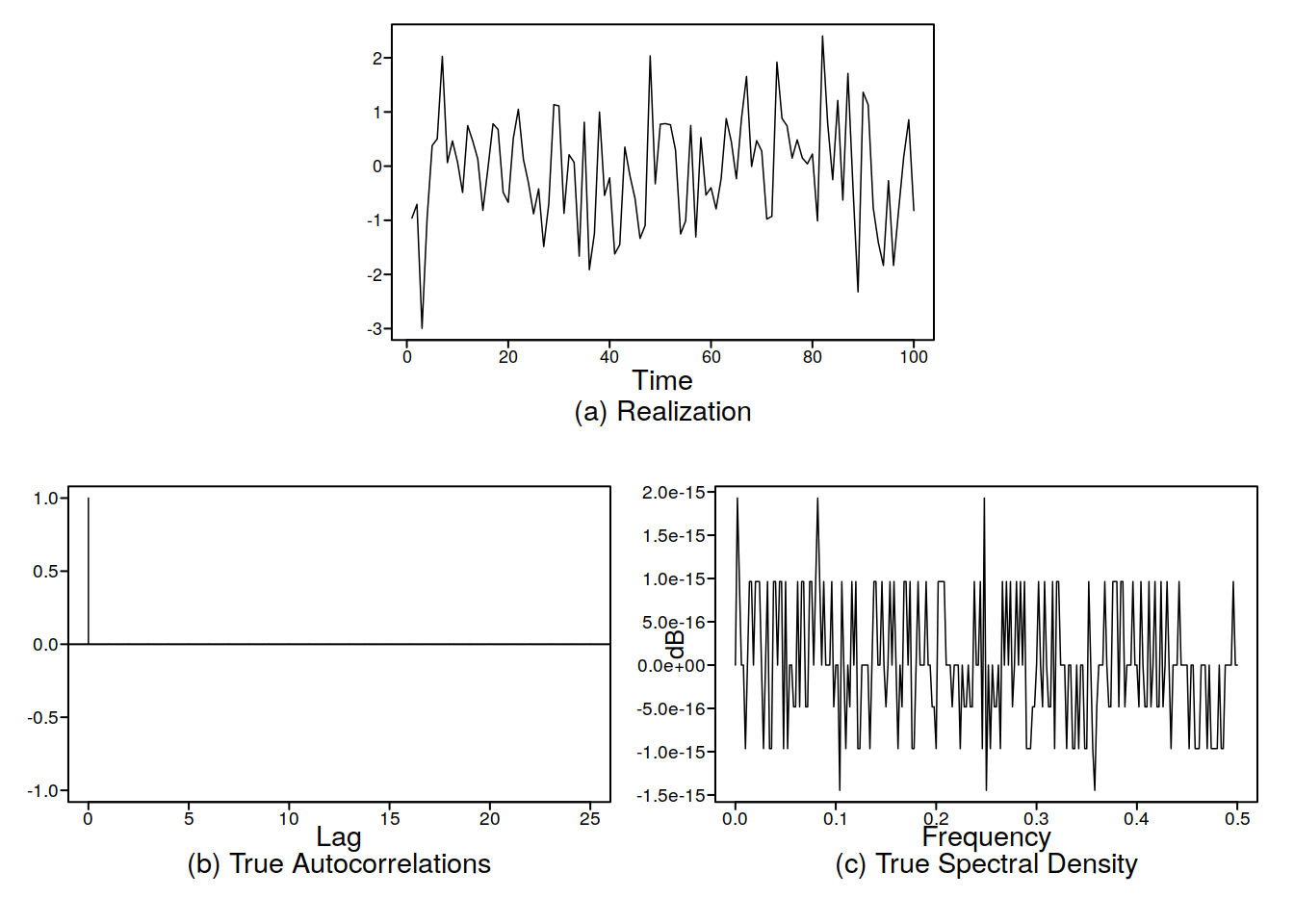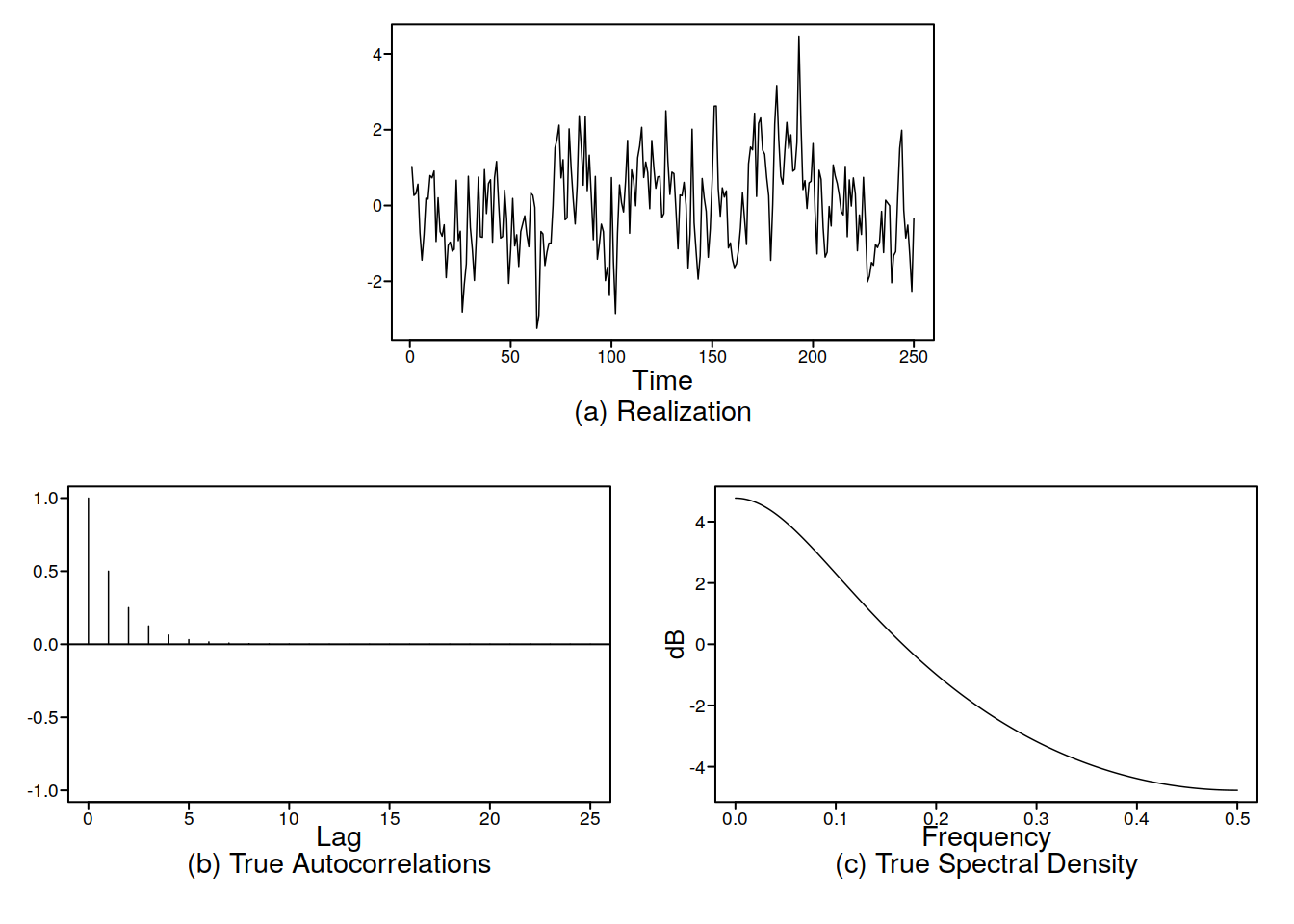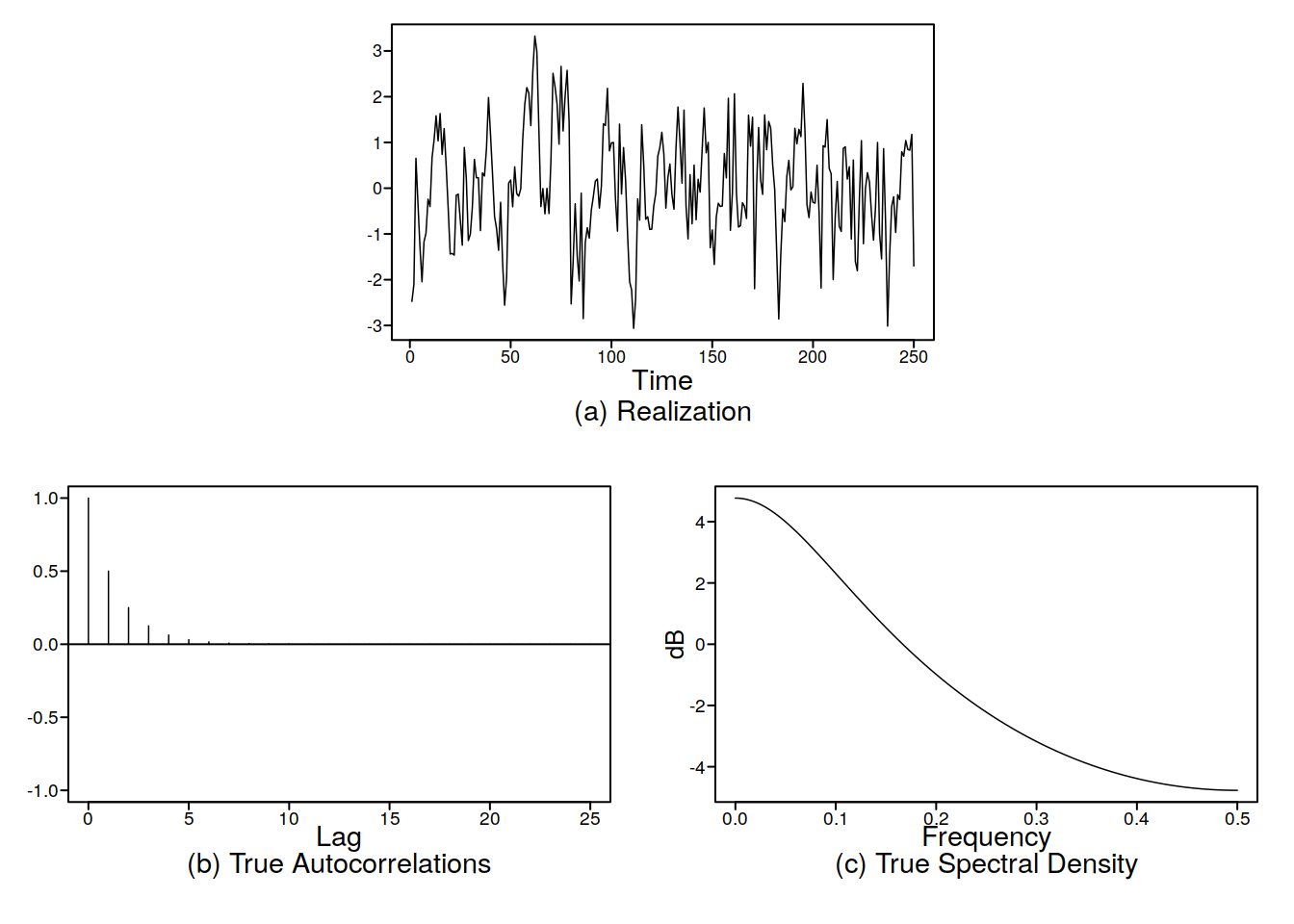# Unit 17 ARMA(p,q)

An ARMA model is an autoregressive moving average model. Written in operator form we have:

$(1-\phi_1 B - ... - \phi_pB^p)X_t = (1 - \theta_1 B - ... - \theta_q B^q)$

If we remember the plotts.true.wge plots we did in a previous section, you may have wondered what happens when the AR and MA chave common factors? Well, they cancel out conveniently! Lets look at an example:

plotts.true.wge(phi = c(1.1, -0.9), theta = c(1.1, -0.9))Wow! It is zero everywhere on the spectral density! BUT, it is not actually zero, as this is a logarithmic scale. This means it is actually one everywhere, and this leads us to the conclusion, along with the ACF and common sense that if all the factors between the AR and MA components are the same, we are left with $$X_t = a_t$$, pure white noise! This allows us to conclude that factors can cancel out, at least with my pea brain.

Another example:

plotts.true.wge(250, phi = c(1.3, -0.4), theta = 0.8)
plotts.true.wge(250, phi = 0.5)Bam! They are the same!!! Wow!